The robot counts Variables Numerical expressions Setting a value to a variable Using variables in a program Summary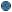Variables The data processed by a program are stored in the computer memory. In order to access the data, the programmer associates a variable with the data. * The variable name is defined by the programmer. This name allows the computer to retrieve the data in its memory. The variable name is like a data address in memory.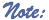A variable name may contain letters and digits but no space and must begin with a letter. Its maximum length is 32 characters. It must be different from the robot language keywords. *The variable value is the content of the memory location referenced by the name. * In RobotProg, the variables are defined for the whole program (main program and all subroutines).Their values are integer. All variables have the value 0 when the program execution begins. * Example : in this chapter, we are going to count the number of steps made by the robot. It will be represented by a variable with the name nSteps.Numerical expressions Numerical expressions are calculus formulas They may contain variables, numbers, parenthesis and operators. The calculus result is an integer. Example : assume the variable named nSteps value is 5 when the expression nSteps + 3 is executed. The expression result will be 8. A numerical expression may also contain numerical functions predefined by means of keywords, like DistanceFromWall : this function returns the number of tiles between the robot and the wall ahead. Example : assume the robot is 4 tiles away from a wall, the expression 2 * DistanceFromWall will return the result 8Setting a value to a variable Setting a value to a variable is done with an assignement instruction like : variable Name = numericalExpression Example : nSteps = DistanceFromWall When the assignment instruction is executed, the expression value is computed, then this value is stored in the variable the name of wich appears at the left of the sign = In a flowchart, the assignment instruction is written inside an assignment block.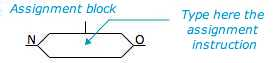The sign = is used in many programming languages for the assignment instruction. It has a different meaning from the sign = used in mathematics. For instance, the instruction nSteps = nSteps + 1 is correct. Assuming nSteps value is 5 before the instruction is executed, when the expression nSteps + 1 is computed, it gives 6 as result, then this value is stored in the variable nSteps : this instruction has increased by one the variable value. It is called an incrementation.Using variables in a program Now, you are going to write a program to find haw many steps were done by the robot. The robot will move to a wall, then turn to move to another wall. The method is easy : for each robot move, we increment the variable nSteps. - Assignment instruction is available at level 4 : so you have to select level 4 or higher. - You may use any ground. - Open a new program and enter the following flowchart :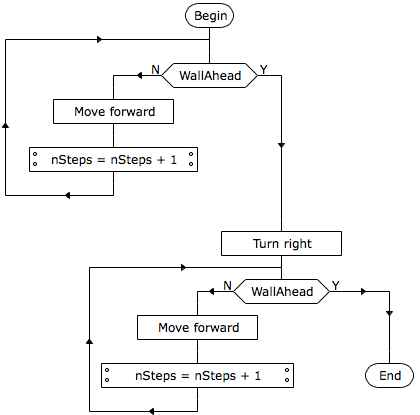- Run the program and click the button "Show variables", so that you can watch the variable nSteps value changing when the robot moves. - Try to run the program for different initial positions of the robot : the program will end with different values of nSteps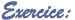Build a program moving the robot like in previous program but with a different way of counting the number of steps, using the keyword DistanceFromWall For the fisrt section, before the robot moves : distance = DistanceFromWall For the second section, just after turn rignt block : distance = distance + DistanceFromWall Summary